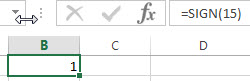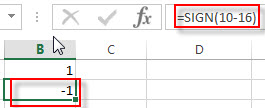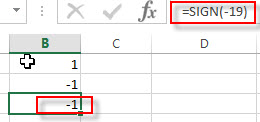## Excel SIGN Function

This post will guide you how to use Excel SIGN function with syntax and examples in Microsoft excel.

### Description

The Excel SIGN function returns the sign of a number. If the number is negative, then the SIGN function will return -1. If the number is equal to 0, then the SIGN function will return 0, and if the number is positive, the SIGN function will return 1. So you can use the SIGN function to get the sign of a given number.

The SIGN function is a build-in function in Microsoft Excel and it is categorized as a Math and Trigonometry Function.

The SIGN function is available in Excel 2016, Excel 2013, Excel 2010, Excel 2007, Excel 2011 for Mac.

### Syntax

The syntax of the SIGN function is as below:

`=SIGN (number)`

Where the SIGN function argument is:

• Number -This is a required argument. The number for which you want to get the sign

Note:

• If the supplied number is non-numeric, The SIGN function will return #VALUE! Error.

### Excel SIGN Function Examples

The below examples will show you how to use Excel SIGN Function to get the sign of a number.

1# to get the sign of a positive number 15, enter the following formula in Cell B1.

`=SIGN(15)`2# to get the sign of the result of 10 minus 16, enter the following formula in Cell B2.

`=SIGN(10-16)`3# to get the sign of a negative number -19, enter the following formula in Cell B3.

`=SIGN(-19)`